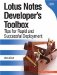# Working with Arrays

Using the DIM statement (which is short for dimension), you can store one or more values in a single variable. This is called an array of values. In its simplest form, the DIM statement is used to create a one-dimensional array that contains one to many fixed values. After the array has been declared, you can assign a value to each element in the array (up to the maximum array size specified) using the element index number. For example:

```Dim theMonth (1 to 12) as String
theMonth (1) = "January"
theMonth (2) = "February"
theMonth (3) = "March"
theMonth (4) = "April"
theMonth (5) = "May"
theMonth (6) = "June"
theMonth (7) = "July"
theMonth (8) = "August"
theMonth (9) = "September"
theMonth (10) = "October"
theMonth (11) = "November"
theMonth (12) = "December"
```

Note

Month is a keyword and cannot be used as an object identifier. Thus, like the previous example, consider appending "the" as an alternative (e.g., theMonth).

A multi-dimensional array is used to store a complex set of values in a single variable. LotusScript currently allows up to eight dimensions. To create a multi-dimensional array, comma-separate each additional array size to the statement. For example:

```Dim holiday (1 to 12, 1 to 31) as String
holiday (1, 1) = "New Years Day"
holiday (2, 14) = "Valentines Day"
holiday (4, 1) = "April Fools Day"
```

Alternatively, you can use the re-dimension (REDIM) statement to resize an array of elements. Using this approach, you can change or redefine the total number of elements that can be stored in the array. However, this approach requires a bit more coding to implement. First, you'll need to create a counter to track the total number of elements in the array (or utilize the Ubound() statement).

Next, you can manage the contents of the array using an IF statement to determine if the array contains any elements. If the counter is set to zero, you'll need to declare the array before you assign a value to the first element in the array. If the counter is greater than zero, the array already contains a value, and you'll need to re-dimension the array. This is accomplished by preserving the current array contents and appending the new value to element list.

```For x = 1 To 5
Redim Preserve myList(x) As String
myList(x) = "This is record number " + x
Messagebox myList(x)
Next
```Lotus Notes Developers Toolbox: Tips for Rapid and Successful Deployment
ISBN: 0132214482
EAN: 2147483647
Year: N/A
Pages: 293
Authors: Mark Elliott